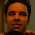## Tuesday, 4 October 2011

### Simple time series plot using R : Part 2

I would like to share my experience of plotting different time series in the same plot for comparison. As an assignment I had to plot the time series of Infant mortality rate(IMR) along with the SOX emission(sulphur emission) for the past 5 decades in the same graph and compare how the intensities have been varying in the past 5 decades.Well to start with there is a problem of how to get these plots in the same graph as one is a mortality rate and other is an emission rate, having different units of measurements!

What we essentially want to do is to see how the intensity of these problem has been changing, whether the intensity has increased/decreased. From a policy makers standpoint, whether it requires immediate attention or not. So what we can do instead is divide all the IMR values by the maximum IMR value that we have for the past 5 decade and store them as "IMR.std". Similarly divide all the SOX values by the maximum SOX value and store it in "SOX.Std". What we have achieved is a parsimonious way of representing the 2 variables that have values between "0 and 1".(Achieving the desired standardization).

Now that we have "IMR.Std." and "SOX.Std." both with values between "0-1" I can plot them in the same graph. Recalling from the previous post

# Make sure the working directory is set to where the file is, in this case "Environment.csv":

# Plotting the "IMR.Std" on a graph

plot(a\$Year, a\$IMR.Std., type="l", xlab= "Years", ylab= "Intensity of problem(normalized between 0-1)", col="green" , lwd=2)

# Adding the plot for "SOX.Std."
# lines(...) command basically adds the argument variable to the existing plot.

lines(a\$Year, a\$SOX.std., type="l", col="black", lwd=2)

Ideally this should have done the job. Giving me the IMR.Std(in green) and SOX.Std.(in red) in the same plot. But this dint happen for the reason that the data for SOX was available only after 1975 and also the data was not available for alternate years. Well I thought that R would treat it trivially and just plot the "non-NA" values of SOX.std. that were there but as it happens that this was not such a trivial thing for R. It demands a lot more rigor(just like a mathematical proof), to execute a command, not taking anything for granted. Hence to get the desired result I had to specify that it considers only the "non-NA" values for SOX.Std.

The code for SOX.std had to be altered a bit:

plot(a\$Year, a\$IMR.Std., type="l", xlab= "Years", ylab= "Intensity of problem(normalized between 0-1)", col="green" , lwd=2)

# All I need to make sure now is that I direct R to refer to only the "non-NA" values in the SOX.Std. variable.
lines( a\$Year[ !is.na(a\$SOX.std.) ], a\$SOX.std.[ !is.na(a\$SOX.std.) ], type="l", col="black", lwd=2)

# If there are 2 separate graphs on the same plot to label the different graphs(depending on their color in this case) we use the legend(...) command :

b <- c("IMR rate", "SOX")
legend("bottomleft", b , cex=0.8, col=c("green", "black"), lwd=2, bty="n")

# title(...) command gets a main title to the plot
title(main="Stage model for country X", col.main="Black", font.main=4)This is how the plot finally looks like.

It was Utkarsh's generosity, who gave me the codes, that saved me a lot of time in solving this small issue, I wish to pass this on as it might save someone else's.

1.Thank you once again, Shreyes, for such a wonderful job (I also thank Utkarsh for his generosity in giving you the codes).

Could you please explain what are:
(i) lwd = 2 in the plot() function and
(ii) cex = 0.8 and bty = n in the legend() command

Also, if I have non-available data (NA) in a time series, should it just be blank in the .csv file or should something special be written there?

Thank you very much.

1.(i) "lwd" is used to specify the line width.
(ii) "cex" A numerical value giving the amount by which plotting text and symbols should be magnified relative to the default. "bty" is specify the type of box required for defining the various lines in the plot.("n" suppresses the box, hence you see there is no box where I have defined the green and black lines in the lower left corner. legend() is to specify what you want to write in that box (in this case the IMR and the SOX emission rate.

(iii) Well it depends on what you want to do with the data. In my case above, I could not plot the data unless I specified to R that I want it to consider only the non-NA values.

~
Shreyes

2.I have taken note. Thank you once again, Shreyes.